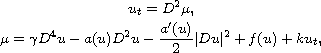Electron. J. Diff. Equ., Vol. 2012 (2012), No. 127, pp. 1-13.

### Optimal control problem for a sixth-order Cahn-Hilliard equation with nonlinear diffusion Changchun Liu, Zhao Wang

Abstract:
In this article, we study the initial-boundary-value problem for a sixth-order Cahn-Hilliard type equationwhich describes the separation properties of oil-water mixtures, when a substance enforcing the mixing of the phases is added. The optimal control of the sixth order Cahn-Hilliard type equation under boundary condition is given and the existence of optimal solution to the sixth order Cahn-Hilliard type equation is proved.

Submitted March 1, 2012. Published August 14, 2012.
Math Subject Classifications: 49J20, 35K35, 35K55.
Key Words: Cahn-Hilliard equation; existence; optimal control; optimal solution.

Show me the PDF file (239 KB), TEX file, and other files for this article.Changchun Liu Department of Mathematics, Jilin University Changchun 130012, China email: liucc@jlu.edu.cn Zhao Wang Department of Mathematics, Jilin University Changchun 130012, China email: wangzhao2717@163.com